In this subsection, the math is fairly simple, but pay very close attention to the geometry described since it will be used as the basis for much of what follows in linear algebra. For binary operations between two vectors, only addition and subtraction are defined. Like vector-scalar operations, vector-vector operations are accomplished by operating on the corresponding entries of each vector individually. So for vector addition of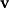and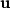we have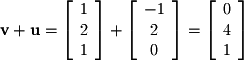Vector/vector subtraction is defined similarly.
Excercise 2-2.
Perform the following operations:
1.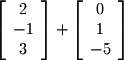2.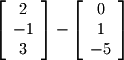Vector addition and subtraction can also be defined geometrically. Vector addition is known as the head-to-tail method because it consists of placing the tail of one vector at the head of the previous vector. In Figure 2-2a we have the following 3 vectors:
Object could not be loaded.
Figure 2-2. Geometry of Vector Addition
Figures 2-2b through 2-2d show the movement of the tail of each successfive vector to the head of the previous vector in order to accomplish the addition.

Subtraction can be thought of in two different ways. If you have a series of vectors as in Figure 2-3a to subtract, the easiest way is to negate the subtracted vectors as shown in Figure 2-3b and then use the head-to-tail method for addition as shown in c and d.
Object could not be loaded.
Figure 2-3. Geometry of Vector Subtraction
The second understanding of vector-vector subtraction will appear many times in linear algebra and is shown in Figure 2-3e. If we imagine moving the starting point of the resultant vector from Figure 2-3d to the tip of subtracted vector, then the resultant vector is the vector between the two original vectors. More formally we can say that given two vectors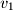and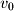, the vector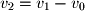is the vector that has its starting point at the head ofand its ending point at the head ofas shown in Figure 2-3e. This is especially useful because it provides a concise way of specifying the start and end points of a vector that does not start at the origin.

From now on in this text, when you see a description such as "consider the vector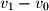" you should normally visualize this as the vector betweenandand not a vector starting at the origin.